# Teaching Fractions with Understanding: Part-whole Concept

##### Age 5 to 14

Published 2011 Revised 2013

In her studies of children learning about rational numbers, Grace Lopez-Charles [see Note 1 below] has identified some different perspectives through which children see fractions. This article starts looking at some of those perspectives, firstly by considering what happens in situations where learners are trying to create equal shares. From sharing using halves learners move to partitioning using other divisions, such as fifths or sixths. This results in the need to develop understandings of equivalence which is discussed in the second section of this article. Finally, we look at some ways of teaching fractions more effectively that arise from Grace's work.

### Equal shares

#### Working with halves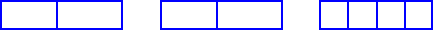This strategy appears to be based upon halves because children are confident with this concept. This approach is more common among younger children, although in her studies Grace has seen many children between the ages of $7$ and $10$ adopting it too. Using this method, the child starts by splitting each region or composite unit into two parts. So, sharing three chocolate bars between four children would be achieved by first halving all the bars. In this case four of the halves can be shared with one whole bar (two halves) remaining. The parts of the third bar are halved again and can now be shared. If a fair share was not possible at this point further halving would take place. At this point a teacher might ask the child "How much has each person got?" and in offering a description the child might reply "Two" because they are counting fragments rather than seeing the equal share as part of a whole.

#### Stuck on counting

In fragmenting the child treats the parts as individual unit items. So, when asked to determine what share each child will receive if three chocolate bars are shared equally among four children, some $7$ year-olds would respond that a child receives two. No conceptual distinction is being made between the 'two' as in number of pieces and the 'two' pieces as fractions of each unit, or the whole (three bars) that is being shared. These children are aware of the fragments as only individual unit items. The complexity here is also whether the 'whole' refers to the individual bars, in which case each child gets three-quarters of the bar, or the 'whole' refers to the three bars. In this latter case the answer would be "A quarter of the chocolate". Here a teacher would be trying to emphasise that we cannot always successfully describe quantity by counting. It would be necessary for portions to be equal in size for counting to offer a way forward.

### Partitioning

Even young children can partition regions or composite units approximately equally among two or three recipients. The older, more mathematically-mature child can partition the region into $x$ number of parts and then designate $y$ of the parts to indicate $\frac{y}{x}$ where $x$ is greater than $2$.

For example: $8$ year-old children were asked to divide a rectangle into eight pieces and then colour two of the pieces red in a diagram similar to the one below.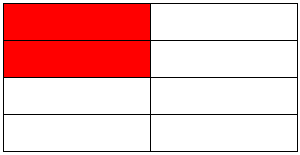The issue here becomes one of equivalence. Grace found that when learners are asked what fraction is unshaded they would say $\frac{6}{8}$. No children in the research identified the coloured part as being $\frac{1}{4}$ of the whole or the uncoloured part as $\frac{3}{4}$. This highlights a question for teachers about how they might raise awareness of those equivalences. This might be achieved through questions inviting learners to describe the same fraction in different ways or by identifying problems with equal answers. For example, in the latter case, sharing $6$ bars of chocolate between $8$ will result in the same amount of chocolate each as sharing $3$ bars between $4$. "Could you get the same answer in a different way?"

In seeing the fraction as a partition and identifying what is the same and what is different about $\frac{3}{4}$ and $\frac{6}{8}$ learners begin to see the fraction as a comparison between the numerator and the denominator (what Grace calls one-to-many or many-to-many comparison). This is based on a ratio concept and Grace argues it also indicates a deeper understanding of fractions. As in the previous example, a child indicating that the uncoloured fraction of the square is three-quarters, is simplifying the denominator and numerator to give an equivalent fraction, without losing the value of the fraction, though some information is lost.

What impact might this have on how we teach children to understand fractions better?

### Ideas for teaching fractions

When teaching fractions in schools, the emphasis is often on situations where the object can easily be cut, folded, split or coloured in equal parts. Although there is some need for this sort of activity, children should be exposed to a wide variety of situations, some where such folding or splitting strategies will not be successful. In experiencing a variety of situations where fractions can be found, learners will have the opportunity to reflect and abstract critical relations in different contextual situations. In other words, children must see a whole in all its representational forms. This will aid them in developing a more robust grasp of the concept of a fraction. Grace describes some of these situations in terms of properties of being discrete or continuous, definite or indefinite (see below). Thus the aim is to engage in working in situations that offer opportunities to explore fractions in each of these situations.

Discrete wholes - sweets, marbles, cherries, beads etc.
Continuous wholes - cakes, chocolate bars, pizzas etc.
Definite wholes - where the extent of the whole is clear, for example: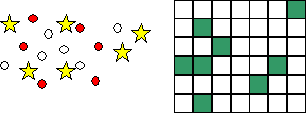Indefinite wholes - where the extent of the whole is not clear, for example we do not know how long the pattern extends in either direction in the image below: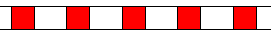In developing a sound understanding of the part-whole concept of fractions, it is necessary for teachers to present situations of fair sharing, where the child is expected to reason out the consequences of different actions. For example with the very young, it might be necessary to begin with a number-free approach;
• Show the children some sweets; question them:- Do you think I have enough sweets that each child will get one sweet?; What will happen if I cut each sweet in half?; Will more or less children get sweets?
• Encourage the children to make meaningful comparisons e.g. '3 pizzas, 4 children', 'does everyone get more or less than half of a pizza? Does everyone get more or less than a whole pizza?'

By presenting the problem qualitatively, teachers are able to generate useful discussions that will encourage the children to use, question and develop their own approaches.

The types of items a teacher sets for individual or group activities should be geared towards developing the fraction concept with the purpose of overcoming some of their difficulties with fractions. By presenting the children with wholes, which are not explicitly divided into equal parts, they are encouraged to analyse the part-whole relationship.

For example:
For each figure, write the fraction shown:
(a)What fraction is K?
(b)What fraction of the figure is not coloured?
(c)What fraction of the whole is missing?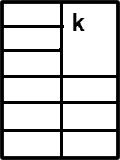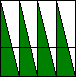Where there is an explicit division of a whole into equal parts, children are able to determine the fraction of the part/parts indicated by counting the number of parts in the whole and the number of parts indicated (double counting). In the figures presented above, it is more difficult for the children to adopt this 'partitioning' approach. Children are required to analyse the relationship of the particular part/parts indicated in relation to the entire whole.

Fractions taught as a part-whole concept, in the manner indicated in this article, can ensure that children have a sound foundation for conceptualising other concepts in fractions. However, it must be noted that despite the wealth of possible examples, an approach to fractions based solely on "part-whole" is too restricted - yielding proper fractions only. Therefore other concepts of fractions need to be explored if children are to have a fuller and better understanding of rational numbers.

Note 1: This article is based on research by Grace Lopez-Charles - Assessment of Children's Understanding of Rational Numbers - PhD Thesis.

### References:

1. Azis, N & Pa, N (1991), Primary school pupils' knowledge of fractions, Journal for Research in Education, 45
2. Nunes, T., (1996), Understanding rational numbers, in T. Nunes and P.E. Bryant (eds.): Children Doing Mathematics. Oxford: Blackwell Publishers.
3. Streefland, L. (1996), Charming fractions or fractions being charmed? In T. Nunes and P.E. Bryant (eds.): How Do Children Learn Mathematics? Hove: Erlbaum.